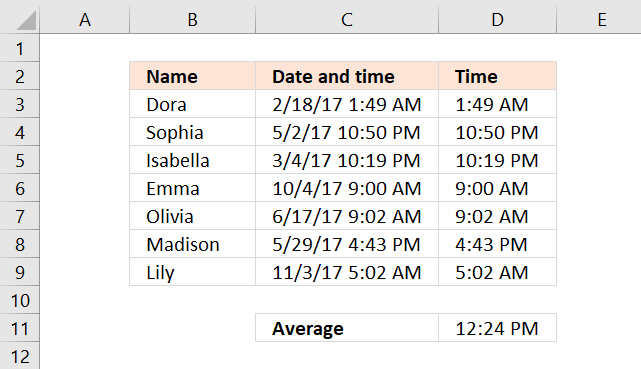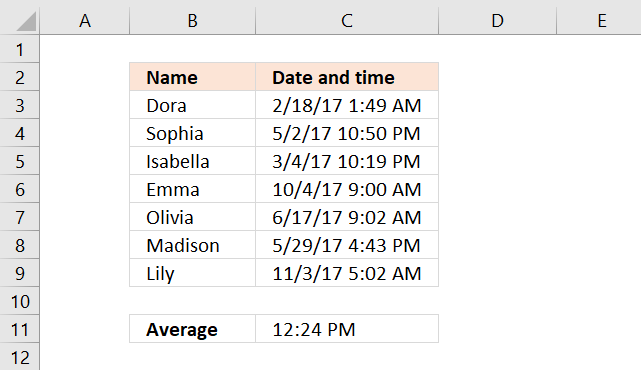Author: Oscar Cronquist Article last updated on March 25, 2018Column C contains both date and time, to calculate the average of only time we need to extract the hours, minutes and seconds from column C. Formula in cell D3:

=TIME(HOUR(C3),MINUTE(C3),SECOND(C3))

Copy cell D3 and paste to cells below. The HOUR function gets the hour from column C, the MINUTE function gets the minute from column C and so on.

The TIME function then builds an Excel timevalue allowing you to calculate the average in cell D11:

=AVERAGE(D3:D9)

Why go through all this mess? The date part in column C is actually a whole number between 1 and 99999. 1 is 1/1/1900 and 1/1/2017 is 42736. If we use the dates in our average calculation the result will come out wrong.

The time part is a number between 0 and 1. For example 01:00 AM is 1/24 or 0.04167, 12:00 PM is 12/24 or 0.5.

### Array formulaYou can combine the calculations in column D by building an array formula, this makes the calculations in D3:D9 unnecessary.

=AVERAGE(TIME(HOUR(C3:C9),MINUTE(C3:C9),SECOND(C3:C9)))

The formula above is an array formula. To enter an array formula, type the formula in a cell then press and hold CTRL + SHIFT simultaneously, now press Enter once. Release all keys.

The formula bar now shows the formula enclosed with curly brackets telling you that you entered the formula successfully. Don't enter the curly brackets yourself.

### Get Excel *.xlsx file

How to AVERAGE time.xlsx